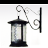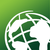# Label Expression Help Needed

206
5
02-13-2014 12:46 PMNew Contributor
I have some pipe data, I used VB Script to display only attributes over 0 (pipe sizes over 0). This is the VB script expression I am
using (the field name for pipe sizes is Diam_in):

Function FindLabel ( [Diam_in], [ ] )
FindLabel = Function FindLabel ([Diam_in])
if ([Diam_in] >= 0) then
FindLabel =  [Diam_in]
else
FindLabel = [ ]
end if
End Function

Question is: How do I add an " to the pipe sizes that display, ie so they label pipes as 8", 12", 10", etc?

Angie
Tags (2)
5 RepliesOccasional Contributor
I have some pipe data, I used VB Script to display only attributes over 0 (pipe sizes over 0). This is the VB script expression I am
using (the field name for pipe sizes is Diam_in):

Function FindLabel ( [Diam_in], [ ] )
FindLabel = Function FindLabel ([Diam_in])
if ([Diam_in] >= 0) then
FindLabel =  [Diam_in]
else
FindLabel = [ ]
end if
End Function

Question is: How do I add an " to the pipe sizes that display, ie so they label pipes as 8", 12", 10", etc?

Angie

Hi there!

You may try doing this on the fourth line hence, FindLabel =  [Diam_in] + "<two single quotes>"byMVP Honored Contributor
The way to do a quote in a sring is with a quadruple quote.  So the line would read:

FindLabel =  [Diam_in] & """"

I have never seen empty brackets passed into a function, so I don't think this is going to work.  Do you really want to label 0" diameters?  What has a diameter that is less than 0"?  If you want to handle Nulls you have to follow the practice in my code below.

So the full code would be:

```Function FindLabel ([Diam_in])
if IsNull([Diam_in]) Then
FindLabel = ""
elseif [Diam_in] >= 0 then ' Consider just > 0
FindLabel = [Diam_in] & """"
else
FindLabel = ""
end if
End Function```New Contributor
It's still not displaying the "; it displays only pipe sizes over 0, so the labels are showing up as 8, 10, etc with no " after the 8, 10, etcbyMVP Honored Contributor
It's still not displaying the "; it displays only pipe sizes over 0, so the labels are showing up as 8, 10, etc with no " after the 8, 10, etc

It works.  I did it before I posted it and it defintely displayed as 8". 10".  What is the code you are trying now?  Are the pipe diameters numeric values or strings?  What font are you using for the labels?

Try a normal expression with just:

[Diam_in] & """"

If that works then you can worry about adding label logic afterward (or write an SQL filter for the labels to avoid having to use the label expression at all).by
Regular Contributor II
```Function FindLabel ([Diam_in])